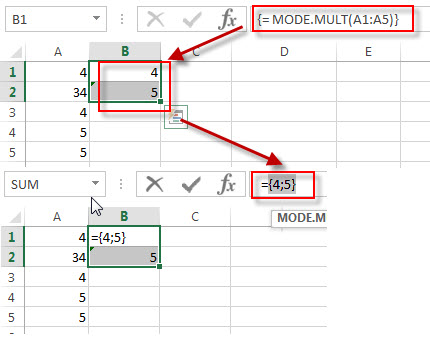# Excel MODE.MULT Function

This post will guide you how to use the MODE.MULT function with syntax and examples in Microsoft excel.

### Description

The Excel MODE.MULT function returns a vertical array of the most frequently occurring number found in an array or range of numbers. If there are two or more frequently occurring numbers in a range of data, the MODE.MULT function will return more than one result, and it is also an array of values, so you need to enter this function into an array formula.

The MODE.MULT function is a build-in function in Microsoft Excel and it is categorized as a Statistical Function.

The MODE.MULT function is available in Excel 2016, Excel 2013, Excel 2010, Excel 2011 for Mac.

### Syntax

The syntax of the MODE.MULT function is as below:

`= MODE.MULT (number1, [number2], ...)`

Where the MODE.MULT function arguments are:

• number1 – This is a required argument.  A number or array or cell reference that contain only numeric values that you want to calculate the mode.
• number2-This is an optional argument.  A number or array or reference that refers to numeric values. It can be up to 255 numeric values.

Note:

• Any arguments can either be numeric values or Defined names, arrays, or cell reference that contain only numeric values.
• If the list of number arguments contain logical values, text, or empty cells, those values will be ignored.
• If the supplied numbers in a range of data do not contain any duplicate numbers, the MODE.MULT function will return the #N/A error.

### Excel MODE.MULT Function Examples

The below examples will show you how to use Excel MODE.MULT Function to the most frequently occurring value in a range of data.

Example 1:  to get a vertical array of the most frequently occurring number found in a range of cells A1:A5 (you need to press CTRL+SHIFT+ENTER to input the below array formula), using the following formula:

`{= MODE.MULT(A1:A5)}`### Related Functions

• Excel MODE Function
The Excel MODE function returns the most frequently occurring number found in an array or range of numbers. If there are two or more frequently occurring numbers in a range of data, it will return the lowest of these numbers.The syntax of the MODE function is as below:= MODE(number1, [number2], …)…
• Excel MODE.SNGL Function
The Excel MODE.SNGL function returns the most frequently occurring number found in an array or range of numbers.The syntax of the MODE.SNGL function is as below:= MODE.SNGL(number1, [number2], …)…
• Excel AVERAGE function
The Excel AVERAGE function returns the average of the numbers that you provided.The syntax of the AVERAGE function is as below:=AVERAGE (number1,[number2],…)….
• Excel MEDIAN Function
The Excel MEDIAN function returns the median of the given numbers. And the median is the number in the middle of a list of supplied numbers.  The syntax of the MEDIAN function is as below:= MEDIAN (number1, [number2], …)…